# OliNo

Renewable Energy

## TC KLV-T8-150 Led tube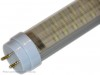presents a 150 cm long led tube. It is one of KLV’s test range. The tubes are in a development phase and will be commercialized in future for the Belgium market. Other measurements will follow to further optimize this range.

This led tube has 396 smd leds and emits a cold white light.

This article shows the measurement results. Many parameters are also found in the Eulumdat file.

See this overview for a comparison with other light bulbs.

### Summary measurement data

parameter meas. result remark
Color temperature 6026 K cold white
Luminous intensity Iv 511 Cd Measured straight underneath the lamp
Beam angle 134 deg 134º for the C0-C180 plane (crossing the length direction of the tube), and 109º for C90-C270 plane (length direction of tube).
Power P 22.1 W
Power Factor 0.97 For every 1 kWh net power consumed, there has been 0.3 kVAhr for reactive power.
Luminous flux 1788 Lm
Luminous efficacy 81 Lm/W
CRI_Ra 63 Color Rendering Index.
Coordinates chromaticity diagram x=0.3207 and y=0.3436
Fitting FL-tube It is connected directly to the grid.
PAR-value 4.2 μMol/s/m2 The number of photons seen by an average plant when it is lit by the light of this light bulb. Value valid at 1 m distance from light bulb.
S/P ratio 1.9 This factor indicates the amount of times more efficient the light of this light bulb is perceived under scotopic circumstances (ow environmental light level).
D x L external dimensions 30 x 1500 mm External dimensions of the lamp. Excluding the pins.
L x W luminous area 1440 x 27 mm Dimensions of the luminous area (used in Eulumdat file). This is equal to the surface at the front on which the leds are mounted.
General remarks The ambient temperature during the whole set of measurements was 25 deg C.  The tube ends of the 120 cm tube are measured on temperature (32 deg C and 42 deg C at the place where the power supply is at).

Warm up effect: during the warm up time the illuminance decreases with ≈7 %.

Voltage dependency: the power consumption and illuminance do not depend significantly on the voltage when it is varied from 200 – 250 V.

Measurement report (PDF)Eulumdat fileRight click on icon and save the file.

### Overview tableThe overview table is explained on the OliNo website. Please note that this overview table makes use of calculations, use this data with care as explained on the OliNo site.

### Eulumdat light diagram

This light diagram below comes from the program Qlumedit, that extracts these diagrams from an Eulumdat file. It is explained on the OliNo site.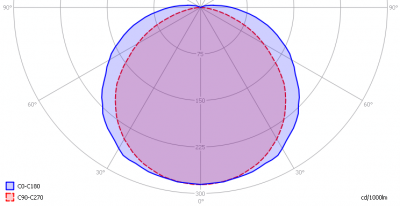The light diagram giving the radiation pattern.

It indicates the luminous intensity around the light bulb. In the length direction (C90-C270) the beam angle is smaller than in the direction perpendicular on the length direction (C0-C180).

### Illuminance Ev at 1 m distance, or luminous intensity Iv

Herewith the plot of the averaged luminous intensity Iv as a function of the inclination angle with the light bulb.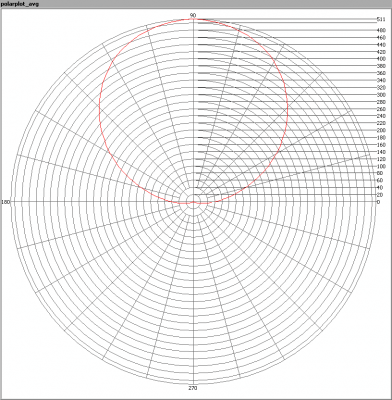The radiation pattern of the light bulb.

This radiation pattern is the average of the light output of the light diagram given earlier. Also, in this graph the luminous intensity is given in Cd.

These averaged values are used (later) to compute the lumen output.Intensity data of every measured turn angle at each inclination angle.

This plot shows per inclination angle the intensity measurement results for each turn angle at that inclination angle. There normally are differences in illuminance values for different turn angles. However for further calculations the averaged values will be used.

When using the average values per inclination angle, the beam angle can be computed, being 109-134º for the C90-C270 and the C0-C180 plane respectively.

### Luminous flux

With the averaged illuminance data at 1 m distance, taken from the graph showing the averaged radiation pattern, it is possible to compute the luminous flux.

The result of this computation for this light spot is a luminous flux of 1788 Lm.

### Luminous efficacy

The luminous flux being 1788 Lm, and the power of the light bulb being 22.1 W, yields a luminous efficacy of 81 Lm/W.

A power factor of 0.97 means that for every 1 kWh net power consumed, a reactive component of 0.3 kVAr was needed.

 Light bulb voltage (used on power supply!) 230 VAC Light bulb current 99 mA Power P 22.1 W Apparent power S 22.8 VA Power factor 0.97

Of this light bulb the voltage across ad the resulting current through it+its constant current driver power supply are measured and graphed. See the OliNo site how this is obtained.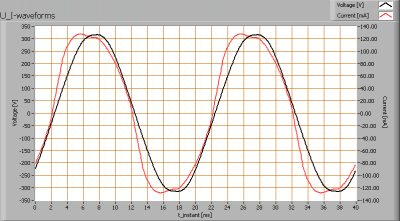Voltage across and current through the lightbulb

The current does quite well resemble a sine. There are some steep edges in the current form, which will lead to higher harmonics. The current phase is leading a little bit the voltage. The result is a nice powerfactor of above 0.95.

Also the power spectrum of the current is determined.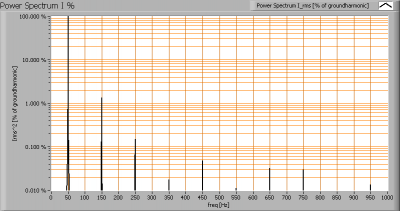Current power spectrum in % of the first harmonic (50 Hz).

Due to some steep edges in the current there are some higher harmonics in the current spectrum.

### Temperature measurements tube

See the 120 cm version of this tube for the temperature images.

### Color temperature and Spectral power distributionThe spectral power distribution of this light bulb, energies on y-axis valid at 1 m distance.

The measured color temperature is about 6025 K which is cold white.

This color temperature is measured straight underneath the light bulb. Below a graph showing the color temperature for different inclination angles.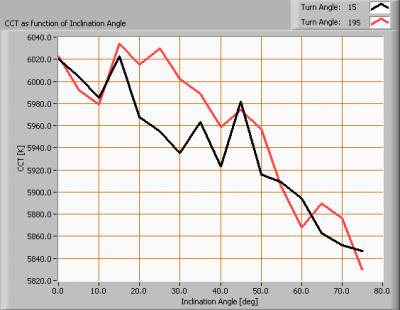Color temperature as a function of inclination angle.

The measurement of CCT has been stopped when the inclination angle was 75º as the illuminance value was decreased to very low values there (< 5 lux).

The beam angle is 134º, meaning an inclination angle of max 67º. Almost all light falls within this angle. The variation of the color temperature within this angle is about 2 %.

### PAR value and PAR spectrum

To make a statement how well the light of this light bulb is for growing plants, the PAR-area needs to be determined. See the OliNo website how this all is determined and the explanation of the graph.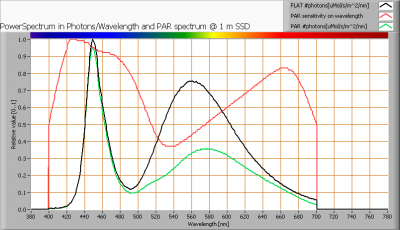The photon spectrum, then the sensitivity curve and as result the final PAR spectrum of the light of this light bulb

The PAR number for this light results in 4.2 μMol/s/m2. This value is valid at 1 m distance from the light bulb and valid roughly inside its beam angle.

The PAR efficiency is 64 % (valid for the PAR wave length range of 400 – 700 nm). So maximally 64 % of the total of photons in the light is effectively used by the average plant (since the plant might not take 100 % of the photons at the frequency where its relative sensitivity is 100 %).

### S/P ratio

The S/P ratio and measurement is explained on the OliNo website. Here the results are given.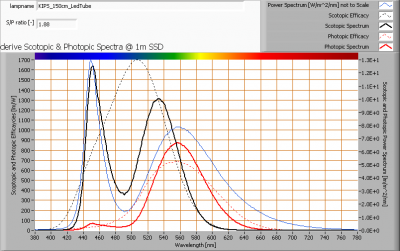The power spectrum, sensitivity curves and resulting scotopic and photopic spectra (spectra energy content defined at 1 m distance).

The S/P ratio is 1.9.

### Chromaticity diagram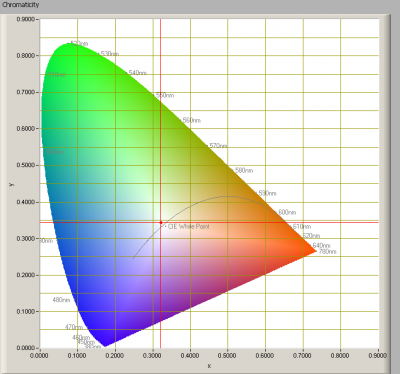The chromaticity space and the position of the lamp’s color coordinates in it.

The light coming from this lamp is at a distance from the Planckian Locus (the black path in the graph).

Its coordinates are x=0.3207 and y=0.3436.

### Color Rendering Index (CRI) or also Ra

Herewith the image showing the CRI as well as how well different colors are represented (rendered). The higher the number, the better the resemblance with the color when a black body radiator would have been used (the sun, or an incandescent lamp).

Each color has an index Rx, and the first 8 indexes (R1 .. R8) are averaged to compute the Ra which is equivalent to the CRI.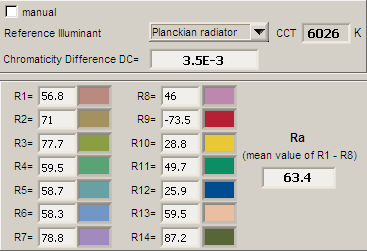CRI of the light of this lightbulb.

The value of 63 is lower than 80 which is considered a minimum value for indoor usage.

Note: the chromaticity difference is 0.0035 indicates the distance to the Planckian Locus. Its value is lower than 0.0054, which means that the calculated CRI result is meaningful.

### Voltage dependency

The dependency of a number of lamp parameters on the lamp voltage is determined. For this, the lamp voltage has been varied and its effect on the following light bulb parameters measured: illuminance E_v [lx], the lamppower P [W], the (Correlated) Color Temperature [K] and the luminous efficacy [Lm/W].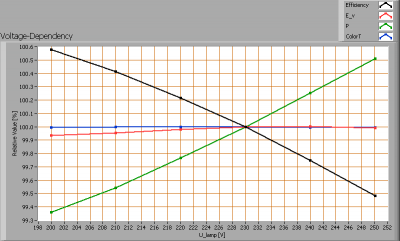Lamp voltage dependencies of certain light bulb parameters, where the value at 230 V is taken as 100 %.

The illuminance and consumed power do not vary significantly the voltage is varied.

When the voltage at 230 V varies with + and – 5 V, then the illuminance varies < 0.1 %, so when abrupt voltage changes occur this effect is not visible in the illuminance output.

### Warm up effects

After switch on of a cold lamp, the effect of heating up of the lamp is measured on illuminance E_v [lx], color temperature CT or correlated color temperature CCT [K], the lamppower P [W] and the luminous efficacy [lm/W].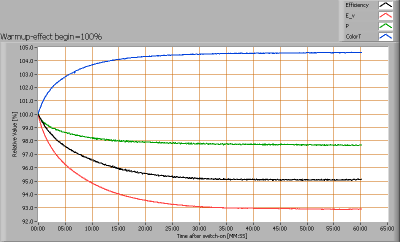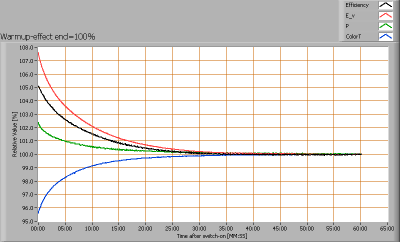Effect of warming up on different light bulb parameters. At top the 100 % level is put at begin, and at bottom at the end.

The warm up time is about 30 minutes. During that time the illuminance decreases with ≈7 % and the color temperature increases with 5 %.